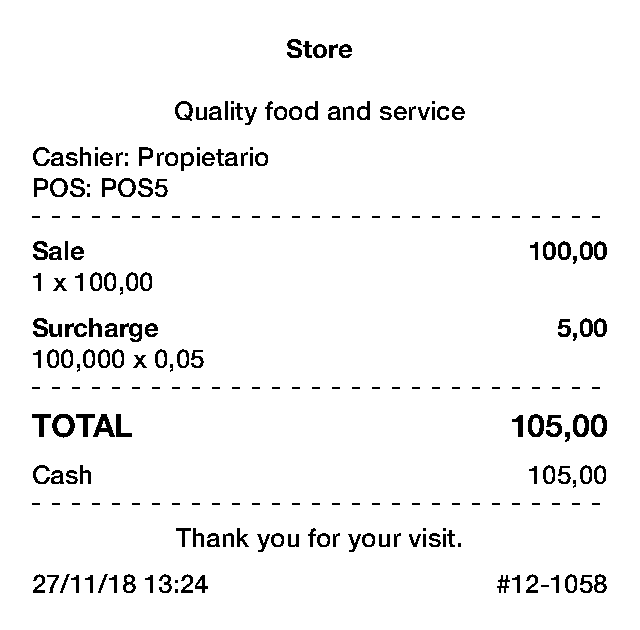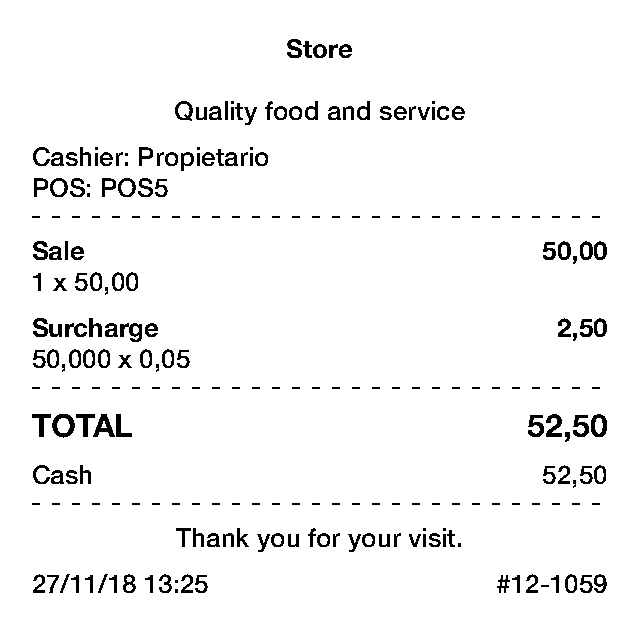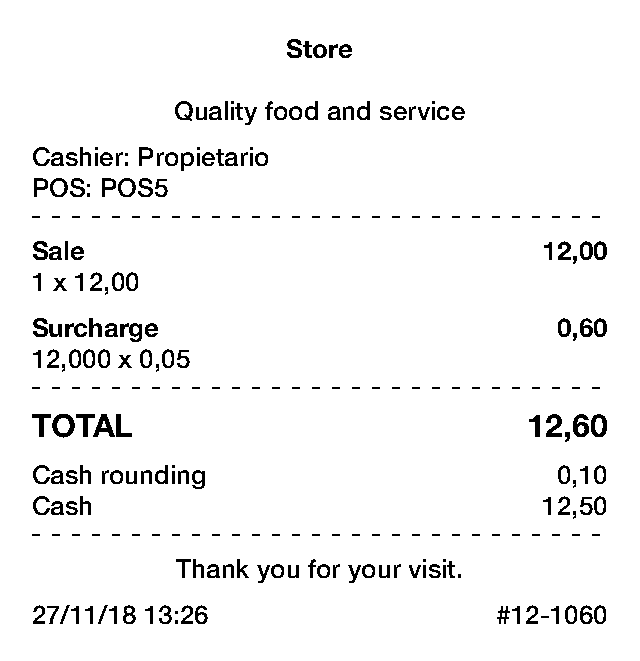There is not an option for surcharges.

I was trying to think of a workaround for this and I think I could find something. What we are going to do is to take leverage of the "Sold by weight" functionality that allows us to calculate the price for an item based on how much it weights, and use it to calculate the surcharge that we want to apply.

Let's say you want to add a surcharge of 5% from the total amount. What we will do is to create a new item, call it "surcharge" and select "sold by weight". In the price field we enter 0.05, and save the item.

Now, everytime you make a new sale, you can add this item to the ticket, and when the app requests to enter a quantity amount you add the sales total. The result is that the app will calculate the 5% of such total as the price of the "surcharge" item and add the item to the ticket. So you will get a new sales total.

This is some examples of how a receipt would look like:In each case, the 5% was calculated correctly.

It would be interesting to hear if you think this is a viable option.

Great solution thank you!